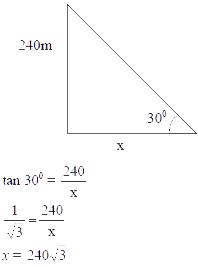# Sample Aptitude Questions of L&T Infotech

1. Type A, 12 kg of rice worth Rs. 40/kg is mixed with Type B, rice worth Rs. 24/kg. What should be the quantity of Type B rice, if the mixture is sold at Rs. 45/kg with 25% profit added in it?
1. 18 Kg
2. 48 Kg
3. 4 Kg
4. can't say

C.P. of the mixture = 45/1.25 = Rs. 36/kg.
So, (40-36)/(36-24) = (N)/(12) ⇒ N = 4 Kg
2. A person spends 1/7th of his salary on travel,1/3rd of the remaining on food, he then spends 1/4th of the remaining on rent. Finally he puts 1/6th of the remaining as a monthly savings, after which he has 25000 left. What is his salary (in Rs.)?
1. 70,000
2. 14,000
3. 84,000
4. 26,000

Let M be the total salary
Therefore, as per question, M × (6/7) × (2/3) × (3/4) × (5/6) = Rs 25000
M = Rs 70000
1. Point C(x,y) divides the distance AB with point A(8,12) and point B(16, 18) in a ratio of 3:5, with AC being shorter than BC. What are the co-ordinates of C?
1. (12,15)
2. (14.5, 12.5)
3. (14.5, 12.5)
4. (11,14.25)

x co-ordinate of C = [(5×8) + (3×16)] / (3+5) = 11
y co-ordinate of C = [(5×12) + (3×18)] / (3+5) = 14.25
Co-ordinates of C is ( 11, 14.25 )
2. How many terms of the sequence -12, -8, -4,…so on, to make a sum of 120?
1. 11
2. 12
3. 10
4. 13

The series is in A.P. where a = -12 and d = 4.
So 120 = (n/2)[2 × (-12) + (n -1) × 4].
On solving this, we get n = 12
3. The value of a machine depreciates from Rs 32,768 to Rs 21,952 in three years. What is the rate % of depreciation?
1. 11%
2. 12.25%
3. 12.5%
4. 33%

Let R be the rate of depriciation, therefore, 21952 = 32768[1-(R/100)]3 . On solving this we get R = 12.5%
4. A TV set listed at Rs 3200 is sold to a retailer at a successive discount of 25% and 15%. The retailer desires a profit of 20%, after allowing a discount of 10% to the customer. At what price should he list the TV set (in Rs.)?
1. 2720
2. 2448
3. 2040
4. 2133

The retailers C.P. = 3200 × 0.75 × 0.85 = Rs 2040.
His expected S.P. = 2040 × 1.2 = Rs 2448.
But S.P. is 90% of the L.P., as there is a discount of 10%.
So L.P. = 2448/0.9 = Rs 2720
5. A student is to answer 10 out of 12 questions in an examination such that he must choose at least 4 from the first five questions. The number of choices available to him is
1. 140
2. 280
3. 196
4. 346

4 questions can be chosen from the first 5 in 5 ways. Remaining 6 questions can be selected from 8 questions in 8C6 ways. So required answer is 8C6 × 5 = 28 × 5 = 140.
6. A rectangular playground with the dimension of 50m X 30 m is surrounded by a 5 m wide road all the sides. What is the area of the road?
1. 600 sq. m.
2. 500 sq. m.
3. 450 sq. m.
4. 900 sq. m.

AREA OF ROAD= area of bigger rectangle - area of smaller rectangle ⇒(60×40)-(50×30)⇒2400-1500=900.
7. It takes 5 sec. for a clock to strike at 5’o clock. If the striking intervals are uniform how much time will it take to strike 9’o clock (in sec.)?
1. 9
2. 10
3. 11
4. 12